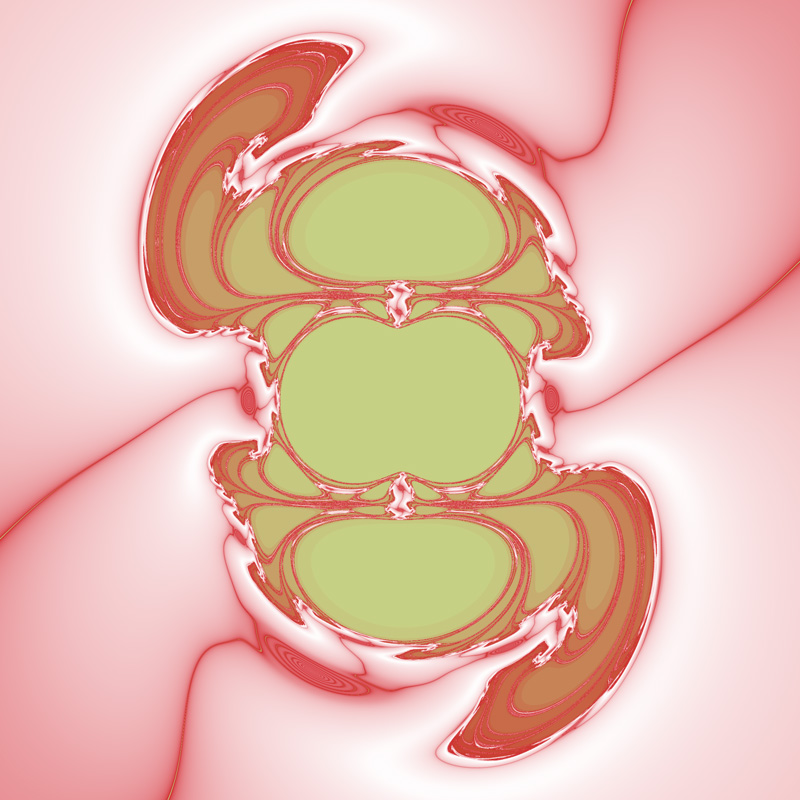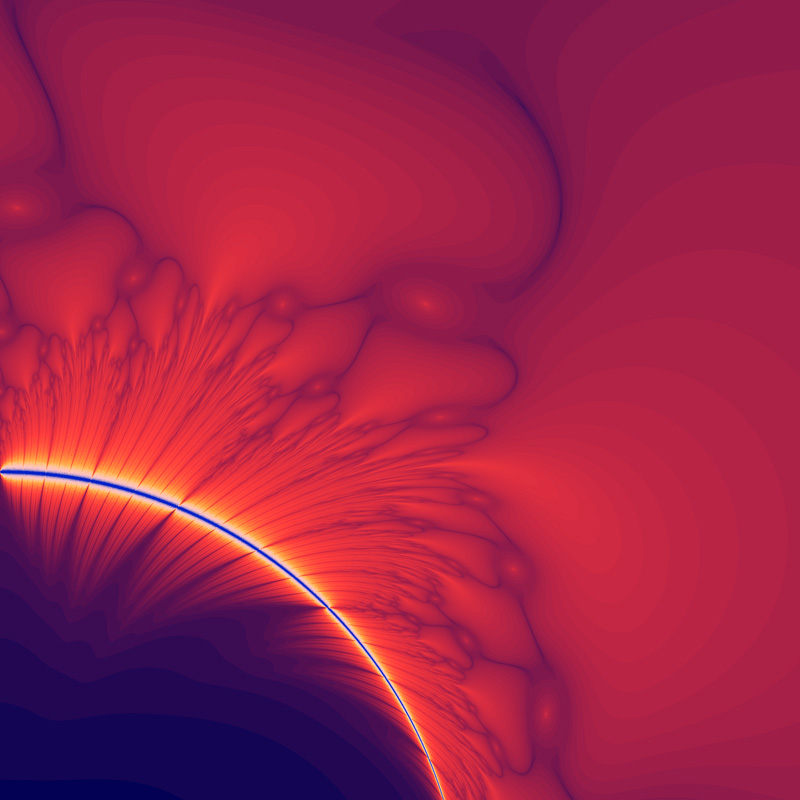# Insideout Dragon

Inspired by Roger Bagula
Written by Paul Bourke
July 2003, updated April 2022

This fractal is calculated in a similar way to the Mandelbrot, that is, a series is calculated and coloured according to how quickly it escapes to infinity. The main difference is that the series in this case is somewhat more complicated. For a coordinate (x0,y0) one forms a complex number z0 = 1 / (x0 + i y0), this becomes the first term of the series.

The series is given by
zn+1 = zn2 + f(zn) + i g(zn)

where

f(r) = r (1 + 2 r + r2) (r2 - 1) / (1 + r3)2

and

g(r) = r (1 - 2 r + r2) (r2 - 1) / (1 + r3)2

Where r is the magnitude of zn.Change denominator from (1 + r3)2 to (1 + r + r7)Change denominator from (1 + r3)2 to (1 + r3)Change denominator from (1 + r3)2 to (1 + r)Change denominator from (1 + r3)2 to (1 + r2)2Change denominator from (1 + r3)2 to (1 + r + r2)